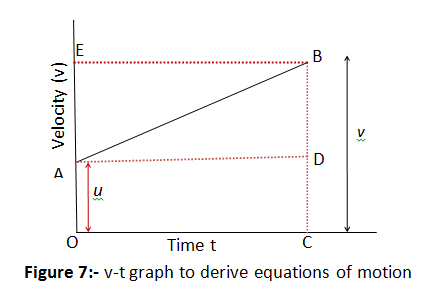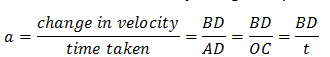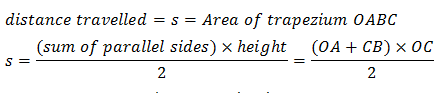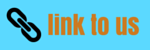# Motion

## 8. Equations of motion by graphical method

• We already know about equations of motion when an object moves along straight line with uniform acceleration. We already know how to derive them but these equations can also be derived by graphical method.

### a. Equation for velocity time relation

• Consider the velocity-time graph of an object that moves under uniform acceleration as shown below in the figure 7.
• From this graph, you can see that initial velocity of the object is $u$ (at point A) and then it increases to $v$ (at point B) in time $t$. The velocity changes at a uniform rate $a$.
• Again from figure it is clear that time $t$ is represented by OC , initial velocity $u$ by OA and final velocity of object after time $t$ by BC.• From graph as given in figure 7 it is clear that $BC = BD + DC = BD + OA$.
So we have
v=BD+u               (1)
• We should now find out the value of BD. From the velocity-time graph (Fig. 7), the acceleration of the object is given bywhich gives, $BD = at$
putting this value of BD in equation 1 we get
$v = u + at$
which is the equation for velocity time relation.

### b. Equation for position time relation

• Let us consider that the object has travelled a distance s in time t under uniform acceleration a. In Fig. 7, the distance travelled by the object is obtained by the area enclosed within OABC under the velocity-time graph AB.
• Thus, the distance s travelled by the object is given by
s = area OABC (which is a trapezium)
s= area of the rectangle OADC + area of the triangle ABD
So,
$s = OA \times OC + \frac{1}{2}\left) {AD \times BD} \right)$
Substituting $OA = u$, $OC = AD = t$ and $BD = at$, we get
$s = \left( {u \times t} \right) + \frac{1}{2} \times \left( {t \times at} \right)$
or,
$s = ut + \frac{1}{2}a{t^2}$
which is the equation of position time relation

### c. Equation for position velocity relation

• Again consider graph in figure 7. We know that distance travelled s by a body in time t is given by the area under line AB which is area of trapezium OABC. So we haveSince $OA+CB=u+v$ and $OC=t$, we thus have
$s = \frac{{\left( {u + v} \right)t}}{2}$
From velocity time relation
$t = \frac{{v - u}}{a}$
putting this t in equation for s we get
$s = \frac{{\left( {u + v} \right)}}{2}\left( {\frac{{v - u}}{a}} \right)$
or we have
${v^2} = {u^2} + 2as$
which is equation for position velocity relation.

## Test your Knowlegde

Fill in the blanks 1) The motion of an object moving  at uniform acceleration can be described with the help of three equations, the equations are _____, ______ and __________
2) The change in the position of an object with time can be represented on the _______ graph.
3) The distance time graph for a body moving with non uniform __________________ is a curve
4) The motion of an object could be uniform or non-uniform depending on whether its velocity is constant or __________________.
5)The product of velocity and time give __________________ of an object moving with uniform velocity.
6) The area enclosed by velocity-time graph and the time axis will be equal to the magnitude of the ________
7) If a body moves with a constant velocity then velocity time gragh  for this body would be _____________ parallel to time axis
8) The acceleration of an object is the change in velocity per __________________time.
9) The value of _____________can be determined using  the velocity time graph.________________ of moving body gives its acceleration.